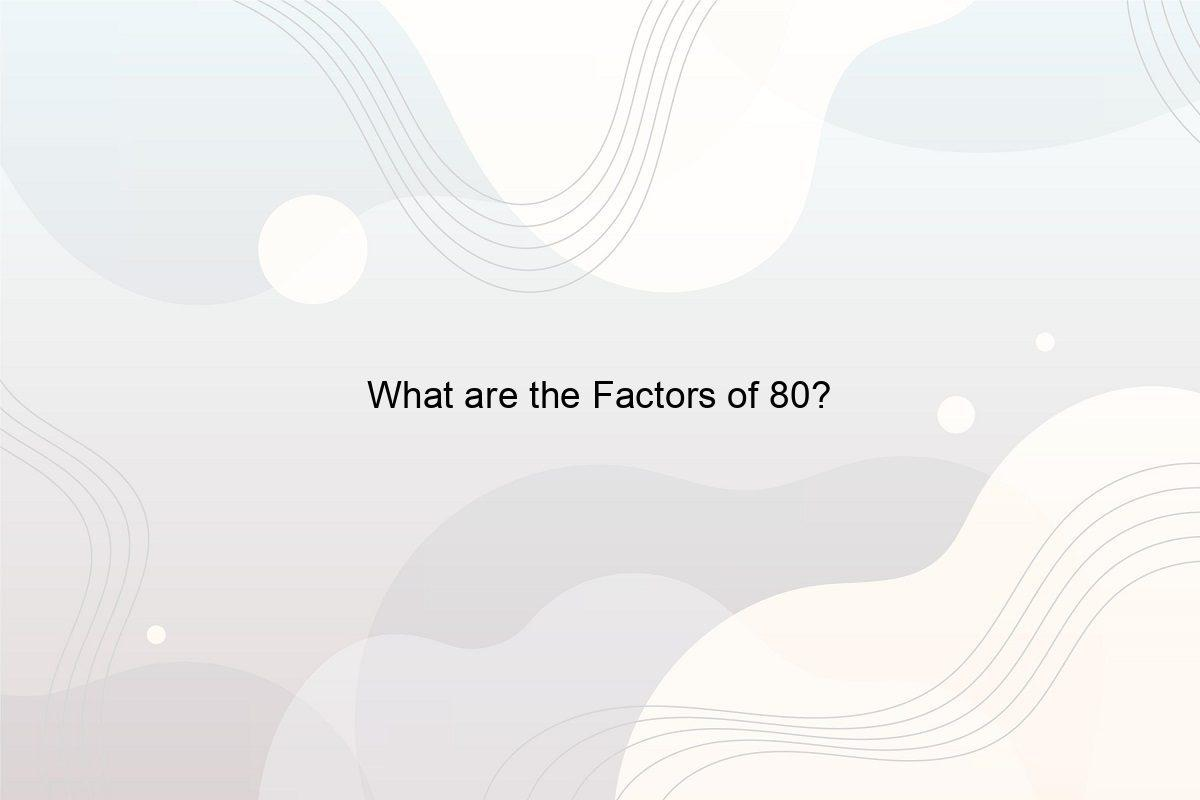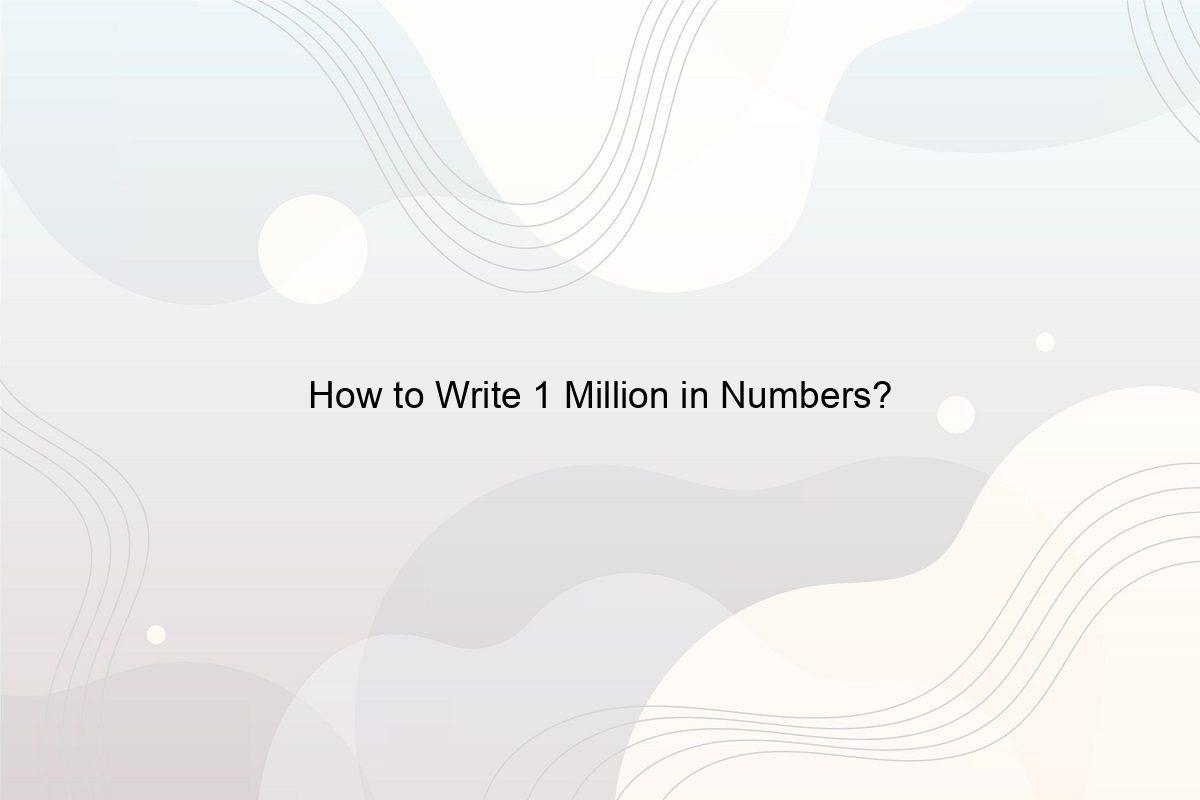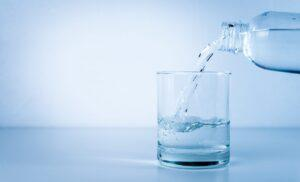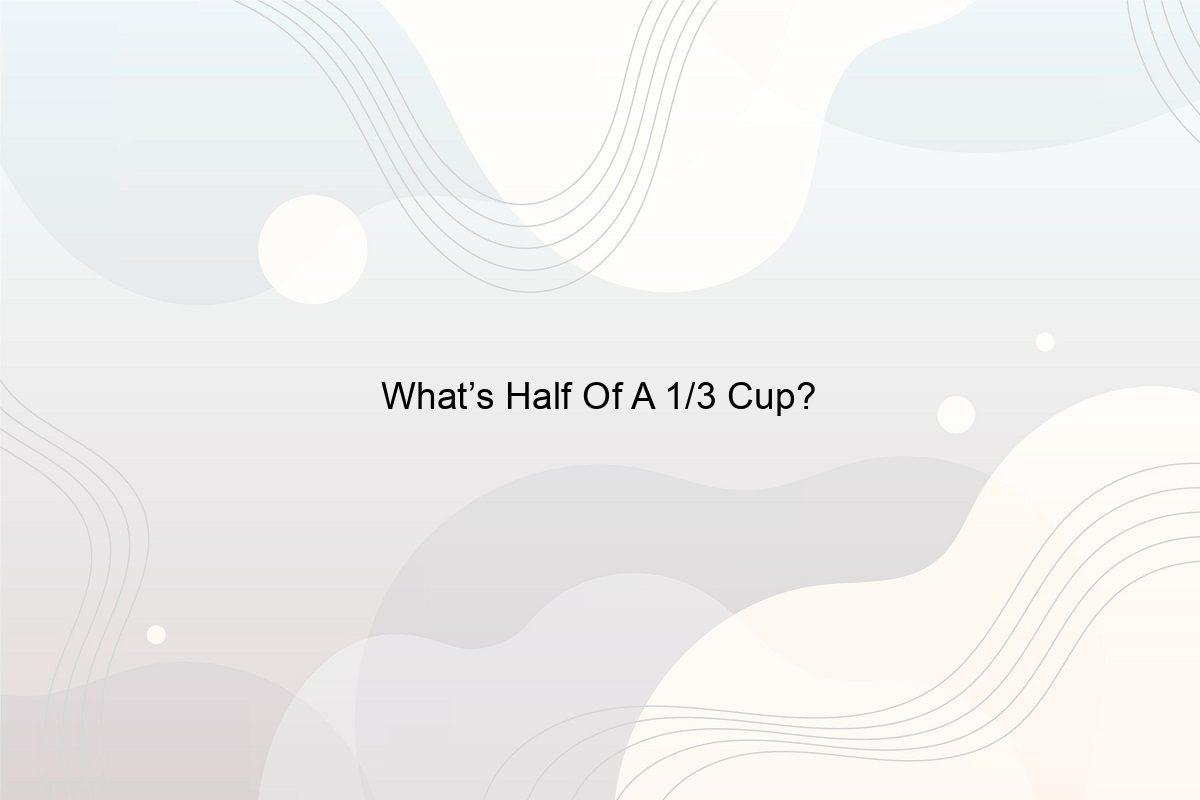﻿ How much is 2 quarts in ounces? - Speeli

# How much is 2 quarts in ounces?

How much is 2 quarts of water in cups? Multiplying the value of quart by 32 will give the result in the number of ounces it has. Hence, the answer here is 64.
1. ## US Customary Measurement System and The Imperial System of measurement

The United States does not use the metric system. The Americans use their native measurement systems which feature units like Gallon, ounce, quart and pint for volumes. However, the same unit, ounce, is used by the British in their Imperial System of Measurement too. The Imperial System of units Is the first set of units defined by the British Weights and Measures Act, 1824. Although much of the modern world uses the metric system of kilometres, kilogram, litres, the US customary system and the Imperial system are also well known.

2. ## Difference between the two

The United States Customary System of measurement has one set of units for dry goods and another for fluids. On the other hand, the Imperial System (followed in the United Kingdom and Northern Ireland) uses a single set of units for all defined measurements. In regular circumstances, the Imperial systems fluid ounce is slightly smaller than the fluid ounce used by the United States customary measurement system. (See How many 8 ounce cups are there in a gallon?)

3. ## The measurements

• 1 United States customary measurement’s fluid quart is equal to 32 fluid ounces
• 1 Imperial quart is equivalent to 38.4 fluid ounces
• 1 United States customary measurement’s ounce is equivalent to 0.03125 United States fluid quart
• 1 United States customary measurement’s ounce is equivalent to 0.0268552188 United States dry quarts
• One Imperial fluid ounce is equivalent to 0.025 imperial quart
4. ## Conversion from one system to another

Since you are now aware of the measurement of the individual fluid measurement systems of the US customary measurement system and the Imperial measurement system, it is also important to know how to convert ounces into a quart. To convert quart into fluid ounce, multiplying the value of quart by 32 will give the result in the number of ounces it has.

Hence, the answer here is 64.

##### Related Posts## What are the Factors of 80?

What are Factors and Multiples? What is Prime Factorisation of Numbers? What are the Factors of 80? What is the LCM of 80?## How to Write 1 Million in Numbers?

How do you Write 1 Million in Numbers? How many Zeros are in a Million, Billion, Trillion, and Quadrillion? What is the Difference between Lakh and Million? How many Millions are 2 Crores?